Courses

# R.C. Mukherjee Test: Gases & Liquids

## 30 Questions MCQ Test Chemistry for JEE | R.C. Mukherjee Test: Gases & Liquids

Description
This mock test of R.C. Mukherjee Test: Gases & Liquids for Class 11 helps you for every Class 11 entrance exam. This contains 30 Multiple Choice Questions for Class 11 R.C. Mukherjee Test: Gases & Liquids (mcq) to study with solutions a complete question bank. The solved questions answers in this R.C. Mukherjee Test: Gases & Liquids quiz give you a good mix of easy questions and tough questions. Class 11 students definitely take this R.C. Mukherjee Test: Gases & Liquids exercise for a better result in the exam. You can find other R.C. Mukherjee Test: Gases & Liquids extra questions, long questions & short questions for Class 11 on EduRev as well by searching above.
QUESTION: 1

Solution:

n,T → const
PV = const

QUESTION: 2

Solution:

n → constant
v → fixed
∴ P & T→ const

QUESTION: 3

### A certain mass of a gas occupies a volume of 2 litres at STP. Keeping the pressure constant at what temperature would the gas occupy a volume of 4 litres -

Solution:

V = 2 litre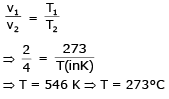QUESTION: 4

If 500 ml of a gas 'A' at 1000 torr and 1000 ml of gas B at 800 torr are placed in a 2L container, the final pressure will be-

Solution: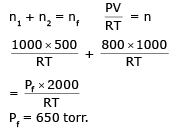QUESTION: 5

Two flasks A and B of 500 ml each are respectively filled with O2 and SO2 at 300 K and 1 atm. pressure. The flasks will contain-

Solution:

n = const.
no of molecules = const
same number of molecules

QUESTION: 6

In the gas equation PV = nRT, the value of universal gas constant would depend only on -

Solution:

R is depend upon unit of measurement

QUESTION: 7

A 0.5 dm3 flask contains gas 'A' and 1 dm3 flask contains gas 'B' at the same temperature. If density of A = 3.0 gm dm-3 and that of B = 1.5 gm dm-3 and the molar mass of A = 1/2 of B, then the ratio of pressure exerted by gases is-

Solution: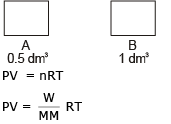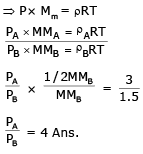QUESTION: 8

When the pressure of 5L of N2 is doubled and its temperature is raised from 300K to 600K, the final volume of the gas would be-

Solution:

PV = nRT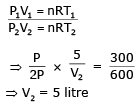QUESTION: 9

If the pressure of a gas contained in a closed vessel in increased by 0.4% when heated by 1°C its initial temperature must be :

Solution: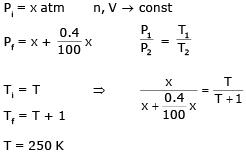QUESTION: 10

A thin ballon filled with air at 47°C has a volume of 3 litre. If on placing it in a cooled room its volume becomes 2.7 litre, the temperature of room is :

Solution: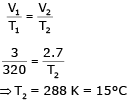QUESTION: 11

If a mixture containing 3 moles of hydrogen and 1 mole of nitrogen is converted completely into ammonia, the ratio of initial and final volume under the same temperature and pressure would be:

Solution: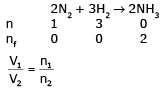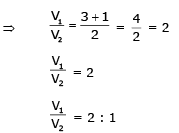QUESTION: 12

SO2 at STP contained in a flask was repleced by O2 under identical conditions of pressure, temperature and volume. Then the weight of O2 will be ______ SO2.

Solution: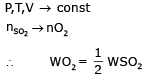QUESTION: 13

Assuming that O2 molecule is spherical in shape with radius 2Å, the percentage of the volume of O2 molecules to the total volume of gas at S.T.P. is :

Solution: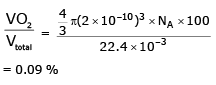QUESTION: 14

Two flasks of equal volume are connected by a narrow tube (of negligible volume) all at 27°C and contain 0.70 mole of H2 at 0.5 atm. One of the flask is then immersed into a bath kept at 127°C, while the other remains at 27°C. The final pressure in each flask is :

Solution:

∴ n1 +n2  = 0.7 moles    (1)
P,V = const.
n1T1 = n2T2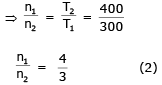n1 = 0.4 & n2 = 0.3
Icontainer PV = n1RT1 (3)
IIcontainer PV = n2RT2 (4)
using (3) & (4)
Final pressure  = 0.5714 atm

QUESTION: 15

Two flasks of equal volume are connected by a narrow tube (of negligible volume) all at 27°C and contain 0.70 moles of H2 at 0.5 atm. One of the flask is then immersed into a bath kept at 127° C, while the other remains at 27°C . The number of moles of H2 in flask 1 and flask 2 are:

Solution: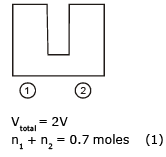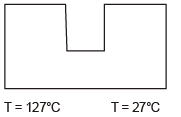P,V  = const
n1T1 = n2T2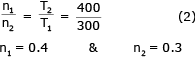QUESTION: 16

A gas is heated from 0°C to 100°C at 0.1 atm pressure .If the initial volume of the gas is 10.0 l, its final volume would be :

Solution: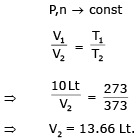QUESTION: 17

Under what conditions will a pure sample of an ideal gas not only exhibit a pressure of 1 atm but also a concentration of 1 mol litre-1. [R = 0.0892 litre atm mol-1 K-1]

Solution: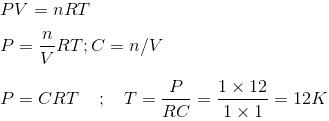QUESTION: 18

A and B are two identical vessels. A contains 15 g ethane at 1atm and 298K. The vessel B contains 75 g of a gas X2 at same temperature and pressure. The vapour density of X2 is :

Solution: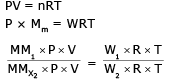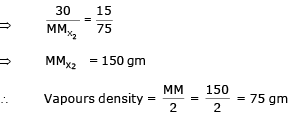QUESTION: 19

The density of neon will be highest at :

Solution:

PV = nRT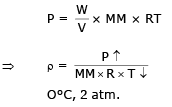QUESTION: 20

A 0.5 dm3 flask contains gas A and 1 dm3 flask contains gas B at the same temperature. If density of A = 3 g/dm3 and that of B = 1.5 g/dmand the molar mass of A = 1/2 of B, the ratio of pressure exerted by gases is :

Solution: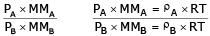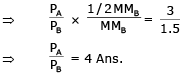QUESTION: 21

A cylinder is filled with a gaseous mixture containing equal masses of CO and N2. The partial pressure ratio is:

Solution: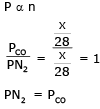QUESTION: 22

A gas X diffuses three times faster than another gas Y the ratio of their densities i.e., Dx : Dy is-

Solution:

Here we use Graham's law of diffusion.

Formula :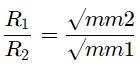Let the rate of gas Y be r1​, then the rate of gas X will be 3r1

Let the density of X and Y be Dx​ and Dy​ respectively then substituting in the formula we have: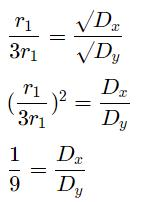The ratio is thus 1:9

QUESTION: 23

At STP, a container has 1 mole of Ar, 2 moles of CO2, 3 moles of O2 and 4 moles of N2. Without changing the total pressure if one mole of O2 is removed, the partial pressure of O2 :

Solution: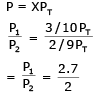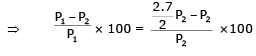⇒ 26 %
Partial pressure of O2 is changed by about 26%

QUESTION: 24

Equal weights of ethane & hydrogen are mixed in an empty container at 25°C, the fraction of the total pressure exerted by hydrogen is :

Solution: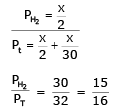QUESTION: 25

A mixture of hydrogen and oxygen at one bar pressure contains 20% by weight of hydrogen. Partical presure of hydrogen will be :

Solution: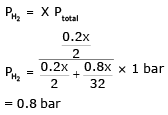QUESTION: 26

A compound exists in the gaseous phase both as monomer (A) and dimer (A2). The atomic mass of A is 48 and molecular mass of A2 is 96. ln an experiment 96 g of the compound was confined in a vessel of volume 33.6 litre and heated to 237°C. The pressure developed if the compound exists as dimer to the extent of 50% by weight under these conditions will be :

Solution:

WA = 48 gm
WA2 → 48 gm
PV = nRT
P ×  33.6 = (nA + nA2) RT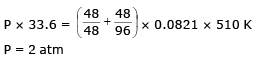QUESTION: 27

Three footballs are respectively filled with nitrogen, hydrogen and helium. If the leaking of the gas occurs with time from the filling hole, then the ratio of the rate of leaking of gases (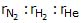) from three footballs (in equal time interval) is :

Solution: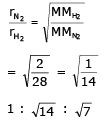QUESTION: 28

The rates of diffusion of SO3, CO2, PCl3 and SO2 are in the following order-

Solution: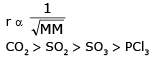QUESTION: 29

20 ℓ of SO2, diffuses through a porous partition in 60 seconds. Volume of O2 diffuse under similar conditions in 30 seconds will be :

Solution: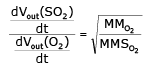QUESTION: 30

See the figure :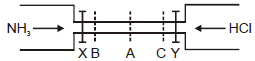The valves of X and Y opened simultaneously. The white fumes of NH4Cl will first form at :

Solution:

According to Graham law of diffusion, rate is inversely proportional to root of molar mass.
Molar massNH3 =17 and Molar massHCl =36.5.
Molar Mass of HCl is more than NH3,  so it rate /speed is less.
So NH4Cl is formed at C because HCl cover less distance and NH3 travel faster and reach at C.